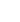30min:
DIABATIC VERSUS ADIABATIC CALCULATION OF TORSION-VIBRATION INTERACTIONS.

JON T. HOUGEN, Sensor Science Division, NIST, Gaithersburg, MD 20899-8441, USA.

The introductory part of this talk will deal briefly with two historical topics: (i) use of the words adiabatic, nonadiabatic, and diabatic in thermodynamics and quantum mechanics, and (ii) application of diabatic and adiabatic ideas to vibrational energy level calculations for a pair of diatomic-molecule potential energy curves exhibiting an avoided crossing. The main part of the talk will be devoted to recent work with Li-Hong Xu and Ron Lees on how ab initio projected frequency calculations for small-amplitude vibrations along the large-amplitude internal rotation path in methanol can best be used to help guide experimental assignments and fits in the IR vibrational spectrum. The three CH stretching vibrations for CH3OH can conveniently be represented as coefficients multiplying three different types of basis vibrations, i.e., as coefficients of: (i) the local mode C-Hi bond displacementsri for hydrogens H1, H2 and H3 of the methyl top, (ii) symmetrized linear combinations of the threeri of species A1 øplus E in the permutation-inversion group G6 = C3v appropriate for methanol, or (iii) symmetrized linear combinations of the threeri of species 2A1 øplus A2 in the permutation-inversion group G6. In this talk, we will focus on diabatic and adiabatic computations for the A1 øplus E basis vibrations of case (ii) above. We will briefly explain how Jahn-Teller-like and Renner-Teller-like torsion-vibration interaction terms occurring in the potential energy expression in the diabatic calculation become torsion-vibration Coriolis interaction terms occurring in the kinetic energy expression of the adiabatic calculations, and also show how, for algebraically solvable parameter choices, the same energy levels are obtained from either calculation. A final conclusion as to which approach is computationally superior for the numerical data given in a quantum chemistry output file has not yet been arrived at.